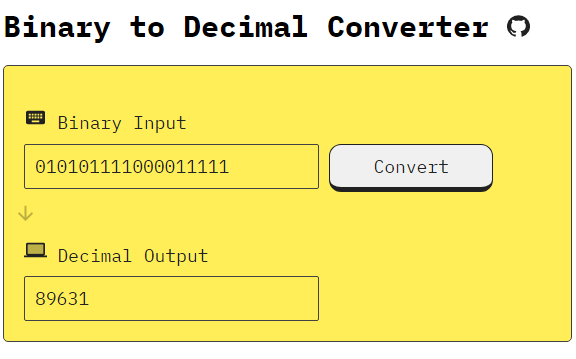/ Calculator

# Binary to Decimal converter using React## konvertilo

In mathematics and digital electronics, a binary number is a number expressed in the base-2 numeral system or binary numeral system, which uses only two symbols: typically "0" (zero) and "1" (one).

The base-2 numeral system is a positional notation with a radix of 2. Each digit is referred to as a bit. Because of its straightforward implementation in digital electronic circuitry using logic gates, the binary system is used by almost all modern computers and computer-based devices.

## Decimal counting

Decimal counting uses the ten symbols 0 through 9. Counting begins with the incremental substitution of the least significant digit (rightmost digit) which is often called the first digit. When the available symbols for this position are exhausted, the least significant digit is reset to 0, and the next digit of higher significance (one position to the left) is incremented (overflow), and incremental substitution of the low-order digit resumes. This method of reset and overflow is repeated for each digit of significance. Counting progresses as follows:

`000, 001, 002, ... 007, 008, 009`, (rightmost digit is reset to zero, and the digit to its left is incremented)
`010, 011, 012`, ...
...
`090, 091, 092, ... 097, 098, 099`, (rightmost two digits are reset to zeroes, and next digit is incremented)
`100, 101, 102`, ...

Binary counting

This counter shows how to count in binary from numbers zero through thirty-one.
Binary counting follows the same procedure, except that only the two symbols 0 and 1 are available. Thus, after a digit reaches 1 in binary, an increment resets it to 0 but also causes an increment of the next digit to the left:

`0000`,
`0001`, (rightmost digit starts over, and next digit is incremented)
`0010, 0011`, (rightmost two digits start over, and next digit is incremented)
`0100, 0101, 0110, 0111`, (rightmost three digits start over, and the next digit is incremented)
`1000, 1001, 1010, 1011, 1100, 1101, 1110, 1111` ...
In the binary system, each digit represents an increasing power of 2, with the rightmost digit representing 20, the next representing 21, then 22, and so on. The value of a binary number is the sum of the powers of 2 represented by each "1" digit. For example, the binary number 100101 is converted to decimal form as follows:

``````1001012 = [ ( 1 ) × 25 ] + [ ( 0 ) × 24 ] + [ ( 0 ) × 23 ] + [ ( 1 ) × 22 ] + [ ( 0 ) × 21 ] + [ ( 1 ) × 20 ]
1001012 = [ 1 × 32 ] + [ 0 × 16 ] + [ 0 × 8 ] + [ 1 × 4 ] + [ 0 × 2 ] + [ 1 × 1 ]
1001012 = 3710
``````